GRE Subject Test: Math : Integration by Parts

Example Questions

Example Question #5 : Integrals

Integrate the following.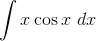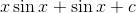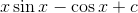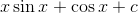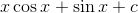Explanation:

Integration by parts follows the formula: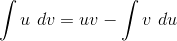So, our substitutions will be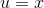and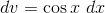which means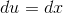and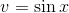Plugging our substitutions into the formula gives us: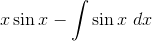Since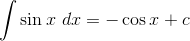, we have: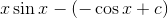, orExample Question #6 : Integrals

Evaluate the following integral.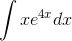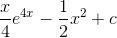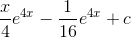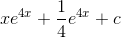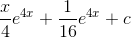Explanation:

Integration by parts follows the formula:In this problem we haveso we'll assign our substitutions:and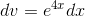which meansand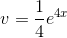Including our substitutions into the formula gives us: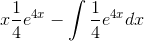We can pull out the fraction from the integral in the second part: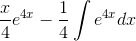Completing the integration gives us: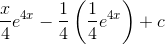Example Question #1 : Integrals

Evaluate the following integral.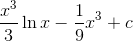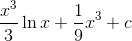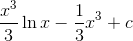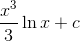Explanation:

Integration by parts follows the formula:Our substitutions will be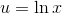and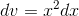which means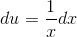and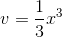.

Plugging our substitutions into the formula gives us: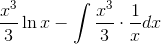Look at the integral: we can pull out theand simplify the remaining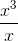as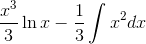.

We now solve the integral: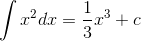, so: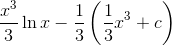Example Question #1 : Integration By Parts

Evaluate the following integral.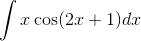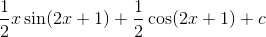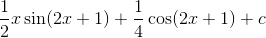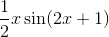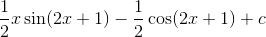Explanation:

Integration by parts follows the formula:.

Our substitutions areand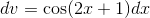which meansand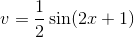.

Plugging in our substitutions into the formula gives us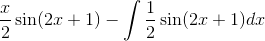We can pulloutside of the integral.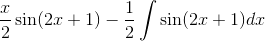Since, we have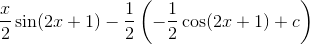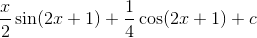All GRE Subject Test: Math Resources